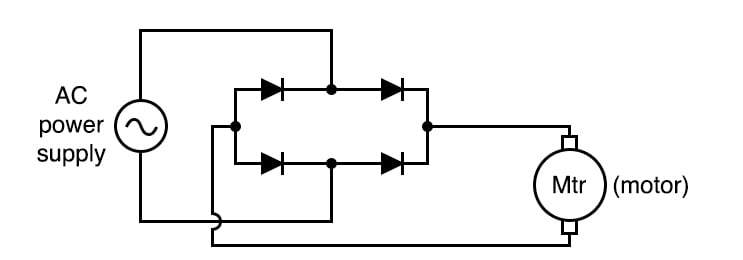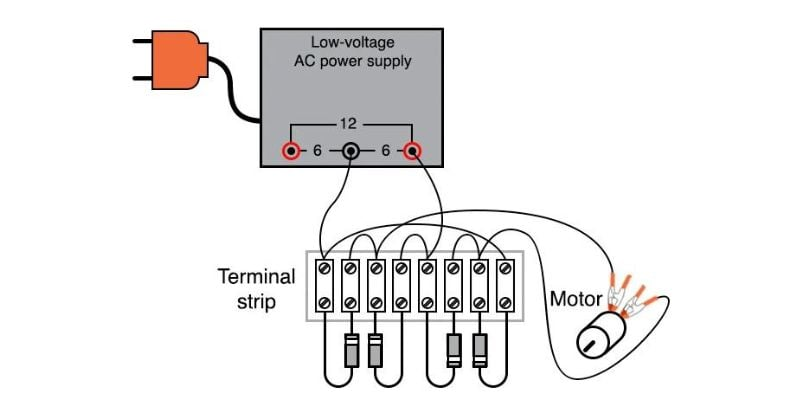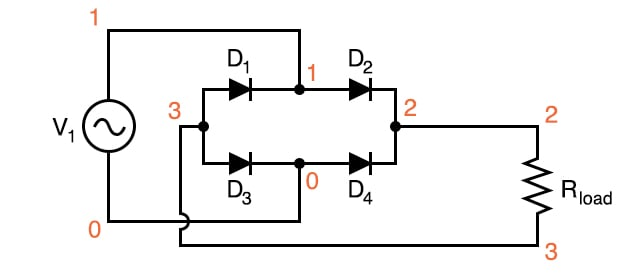Vol. DIY Electronics Projects
Chapter 5 Discrete Semiconductor Circuit Projects

# Si Lab - Full-wave Bridge Rectifier

## In this hands-on semiconductor electronics experiment, build a full-wave bridge rectifier using silicon diodes and learn some of this circuit's advantages and disadvantages.

### Project Overview

The circuit of Figure 1 uses four diodes to provide full-wave rectification without the necessity of a center-tapped transformer##### Figure 1. Schematic diagram of the full-wave bridge rectifier circuit driving a motor

Can you trace the current path from the AC power supply through one diode, through the motor, through a second diode, and back to the AC power supply for both the positive and negative half-cycles of the AC supply voltage?

In applications where a center-tapped or split-phase source is unavailable, this is the only practical method of full-wave rectification. The advantages of the circuit include its simple design and low-cost components.

However, in addition to requiring more diodes than the center-tap circuit, the full-wave bridge suffers a slight performance disadvantage as well. The additional voltage drop is caused by the current having to go through two diodes in each half-cycle rather than through only one.

### Parts and Materials

• Low-voltage AC power supply (6 V output)
• Four 1N4001 rectifying diodes
• Small hobby motor, permanent-magnet type

### Learning Objectives

• Design of a bridge rectifier circuit
• Advantages and disadvantages of the bridge rectifier circuit, compared to the center-tap circuit

### Instructions

Step 1: Build the full-wave bridge rectifier circuit using the four silicon diodes, as illustrated in Figures 1 and 2.##### Figure 2. Terminal strip implementation of the full-wave bridge rectifier circuit driving a motor.

Step 2: Measure the DC voltage across the motor. Compare the DC voltage reading across the motor terminals with the reading obtained from the half-wave rectifier experiment, given the same AC power supply and the same motor. With a low-voltage source such as the one you’re using (6 V RMS or root mean square), the disadvantage of the two voltage drops for this full-wave bridge rectifier is easily measured.

### SPICE Simulation of the Full-wave Bridge Rectifier Circuit

We can simulate the full-wave bridge rectifier using the circuit with node numbers, as illustrated in Figure 3.##### Figure 3. SPICE circuit schematic for a full-wave bridge rectifier circuit

Netlist (make a text file containing the following text, verbatim):

Fullwave bridge rectifier
v1 1 0 sin(0 8.485 60 0 0)
d1 3 1 mod1
d2 1 2 mod1
d3 3 0 mod1
d4 0 2 mod1
.model mod1 d
.tran .5m 25m
.plot tran v(1,0) v(2,3)
.end 

This simulation plots the input voltage as a sine wave that oscillates both positively and negatively. The output voltage is a series of positive humps corresponding to both the positive and negative half-cycles of the AC source voltage. The AC source is set to an 8.485 V peak corresponding to a 6 V RMS sine-wave voltage.

### Related Content

Calculators:

Textbook:

Worksheets: